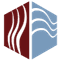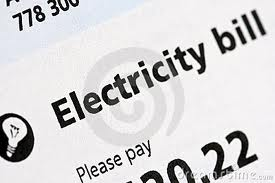# Electric Bills & Air ConditioningAllThingsHVAC
Last updated:
September 25, 2013

How Much Of Your Electric Bills Is The A/C Unit?
By Betty StephensDo you ever wonder how much it costs to run your air conditioning (A/C) unit? There are simple calculations that can help you decide how much your AC is costing. People are always surprised to see how much their monthly electricity costs. Here is an example of the cost to operate an in-room air-conditioning unit for a day and for a month. In general, the higher the BTU rating, the more electricity your unit pulls. And, of course, the energy draw varies by make and model.
The average air conditioner in the U.S. today is approximately 3 tons with a 10 SEER efficiency rating. A unit this size draws approximately 2.94kW per hour. To get the approximate cost to run per hour, you simply multiply this number by the cost of electricity per kilowatt hour. In the San Diego area, electricity costs can vary a great deal depending on the time of day that you are using your air conditioning system—from .147 cents per kilowatt hour at baseline costs to more than .29 cents per kilowatt hour at peak usage. For the sake of convenience we’ll simply use the baseline energy cost of .147 cents per kilowatt hour. Now the math:
2.94 x .147=.432 cents per hour during standard demand or roughly double during peak demand. Assuming 1,200 summer cooling hours, your total cost to run a 3 ton 10 SEER AC for a year can range between \$516 and \$1,023.   If your air conditioner has a SEER rating below the 10 SEER used in this example, your air conditioning costs will be higher. For example, if you have an 8 SEER air conditioner, your system is 20 percent less efficient than a 10 SEER unit, so your cooling costs will be approximately 20 percent higher. If the air conditioner in a home or business is less than 10 SEER, it could be costing hundreds—even thousands of dollars annually.
Understanding Kilowatt-Hours
The kilowatt-hour (kWh) is the standard unit of measurement for electricity in the United States. This is 1,000 watts of electricity being used for one hour. To calculate how much your air conditioner costs to run for an hour, look for its wattage on the box or in the instruction manual and multiply this by the number of hours you use it each day. For example, a 1,000-watt air conditioner running for one hour consumes 1 kWh. By comparison, a 60-watt light bulb running for an hour uses 0.06 kWh (60 watts divided by 1,000). The cost of electricity varies by region. While the average cost in the US in 2011   was 12 cents per kWh, it was as high as 33 cents in Hawaii and as low as 8 cents in Idaho. Check your electric bill to determine your cost.

Calculating Electricity Costs for A/C
To calculate your window air conditioner's energy use, divide the BTUs by the SEER rating. This gives you the watts per hour. Divide that by 1,000 to get kilowatt hours (kWh), which is probably how your electric company calculates your energy bill. You can then multiply the kWh by your power company rate to learn your costs per hour. For example, a 12,000 BTU air conditioner with a SEER rating of 10 and a cost of 25 cents per kWh would calculate as follows:
12,000 BTUs / SEER 10 = 1,200 W = 1.2 kWh
1.2 kWh x \$0.25 = \$0.30 per hour to run your window air conditioner. At 8 hours a day for 125 days of summer, your window air conditioner will have an annual running cost of \$300 a year.
Cutting Costs
A programmable thermostat can reduce the cost of central air conditioning if you program it at a higher temperature while you are at work and while you are sleeping. The temperature you set the air conditioning at should be relative to the outside temperature. If the temperature outside is 90 degrees, setting the air conditioning to 78 should feel quite comfortable in comparison. Drawing the blinds during the day and opening windows at night when it is cool can also save you money. When purchasing an air conditioner, look for Energy Star units, which use up to 14 percent less energy than government requirements and may entitle you to a tax credit of up to 30 percent of its cost.### All Things HVAC

We formed this web site so consumers could have an educational place to go for good, solid information.  We are not trying to sell you anything.

### HOME